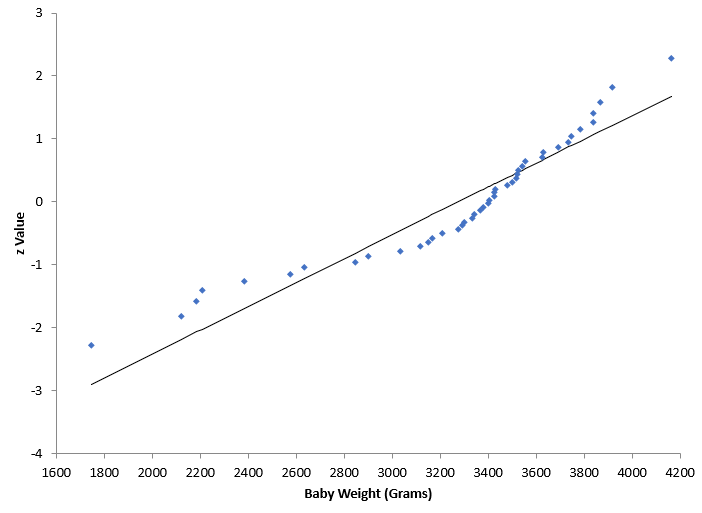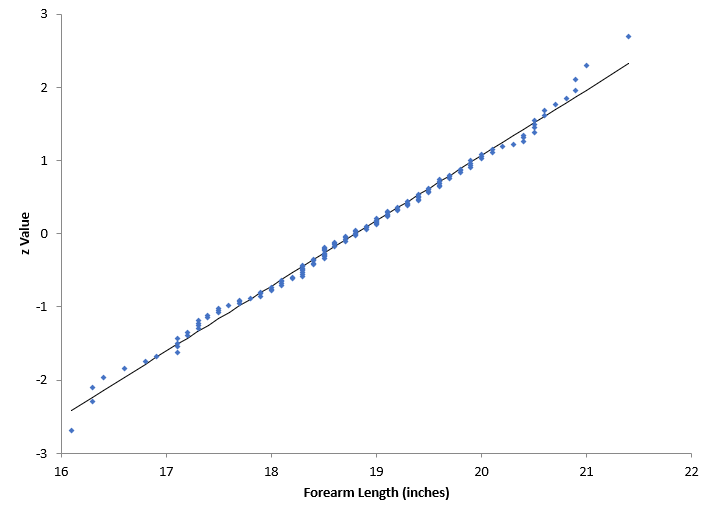## Part 2: Are My Data Normally Distributed?

Our last blog - Are My Data Normally Distributed?- involved using a normal probability plot to answer that question. Essentially, if the data fell along a straight line, we would conclude that the data came from a normal distribution. If it did not fall along a straight line, we would conclude that the data did not come from a normal distribution.## Are My Data Normally Distributed?

You have a set of data. You would like to know if the data comes from a normal distribution. How do you do that? A normal probability plot can be used to determine if sets of data come from a normal distribution. This involves using the probability properties of the normal distribution.  The data are compared to a normal distribution in such a way that will result in a straight line if the data are normally distributed.

#### SPC Knowledge BaseSign up for our FREE monthly publication featuring SPC techniques and other statistical topics.